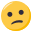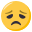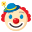# functions+globals work. Closures not.

• Hi folks,
I have a problem with closures. I changed my normal callback functions to closures. The previous version worked fine. Now I get random results for the first number.Output examples:

[martin@laptop Arbeitsfläche]\$ ./a.out
It's a number: -1409639466
It's a number: 5113
It's a number: 774
It's a number: -344
unexpected line end after row: 2 col 13
[martin@laptop Arbeitsfläche]\$ ./a.out
It's a number: 1248173014
It's a number: 5113
It's a number: 774
It's a number: -344
unexpected line end after row: 2 col 13
[martin@laptop Arbeitsfläche]\$ ./a.out
It's a number: 318872534
It's a number: 5113
It's a number: 774
It's a number: -344
unexpected line end after row: 2 col 13

I wonder if I miss something. As I said before. It worked fine without closures with global variables and normal functions. I looks like the variables aren't initialized properly. After the first call of show_number it runs fine.

The test program:

``````node::reference create_number_parser(){
int number;
bool negative;

node::callback init = [&](int c)->void{
number = 0;
negative = false;
};

node::callback show_number = [&](int c)->void{
if(negative) number *= -1;
std::cout << "It's a number: " << number << std::endl;
number = 0;
negative = false;
};

node::callback push_digit = [&](int c)->void{
number = number ? number * 10 + c - '0' : c - '0';
};

node::callback set_sign = [&](int c)->void{
negative = true;
};

node::reference whitespace = node::create_cycle(" \t\n");
node::reference whitespace_after_number = node::create(show_number);
node::reference eof_after_number = node::create(show_number);
node::reference digit = node::create_cycle(push_digit, "0123456789");
node::reference positive_number = node::create();
node::reference negative_number = node::create(set_sign);
node::reference first = node::create(init);

return first;
}

int main(){
try{
node::reference number_parser = create_number_parser();
number_parser->parse_string(" 1234 \n 5113  \t\n +774  -344 +");
}catch(std::exception &e){
std::cerr << e.what() << std::endl;
}

return 0;
}
``````

the previously working version without closures:

``````int number;
bool negative;

void init(int c){
number = 0;
negative = false;
}

void push_digit(int c){
number = number ? number * 10 + c - '0' : c - '0';
}

void show_number(int c){
if(negative) number *= -1;
std::cout << "It's a number: " << number << std::endl;
number = 0;
negative = false;
}

void set_sign(int c){
negative = true;
}

int main(){
node::reference whitespace = node::create_cycle(" \t\n");
node::reference whitespace_after_number = node::create(show_number);
node::reference eof_after_number = node::create(show_number);
node::reference digit = node::create_cycle(push_digit, "0123456789");
node::reference positive_number = node::create();
node::reference negative_number = node::create(set_sign);
node::reference first = node::create(init);

try{
first->parse_string(" 1234 \n 5113  \t\n +774  -344 +");
}catch(std::exception &e){
std::cerr << e.what() << std::endl;
}

return 0;
}
``````

• global variables are initialized to zero by default, local variables get an undefined value when you don't spezify an initialisation value. And for your code, I'm not sure whether `number` and `negative` get a definite value before their first use.

• There're dangling references in your code. The short version is:

``````#include <functional>
#include <iostream>

std::function<int()> dings()
{
int i_am_short_lived = 42;
return [&]{return i_am_short_lived;}
} //      ^
// capture by reference !!!

int main()
{
auto x = dings();
std::cout << x() << std::endl; // Ouch!
}
``````

The solution with global variables is not really much better. "Global state" is almost always a bad thing.

Try something like this:

``````#include <functional>
#include <iostream>
#include <memory>

using namespace std;

function<int()> dings()
{
struct state {
int blah;
};
auto sps = make_shared<state>();
sps->blah = 42;
return [sps]{return sps->blah;}
} //       ^
// capture shared_ptr BY VALUE (not by reference!)

int main()
{
auto x = dings();
cout << x() << endl; // OK!
}
``````

(I didn't test any of that)

• But I thought Closures preserve the local variables? I used them in python.

``````def bla():
val = 5;
def blub():
return val;
return blub;
``````

or something similar. I'm not sure. It's bin a while.

I did a test.

``````node::callback init = [&](int c)->void{
std::cout << "number and negative before initialization: " << number << " " << negative << std::endl;
std::cout << "init is called" << std::endl;
number = 0;
negative = false;
std::cout << "number and negative after initialization: " << number << " " << negative << std::endl;
};

node::callback show_number = [&](int c)->void{
std::cout << "show_number is called" << std::endl;

if(negative) number *= -1;
std::cout << "It's a number: " << number << std::endl;
number = 0;
negative = false;
};
``````

result:

[martin@laptop Arbeitsfläche]\$ ./a.out
number and negative before initialization: 1251359060 74
init is called
number and negative after initialization: 0 0
show_number is called
It's a number: -307192874
show_number is called
It's a number: 5113
show_number is called
It's a number: 774
show_number is called
It's a number: -344
unexpected line end after row: 2 col 13

So init is doing its job. But where is it stored? So, the other correct results are only an accident?

• OK. So here is a working python example. I would like to have a behaviour like this in C++.

``````>>> def outer():
...     var = 5
...     def inner():
...             return var
...     return inner
...
>>> outer()()
``````

• Oh. The result is missing.

Well, ... it's 5.

• But I thought Closures preserve the local variables?

C++ Closures capture things by value (copying) or by reference (storing a reference only). They don't magically extend a local object's life time. Apart from Lambdas being syntactic sugar, there is no other compiler magic going on. If you need to allocate some things dynamically (as in dynamic life-time), you have to say so explicitly (see make_shared, for example).

I don't know Python. What does this snippet do w.r.t. "the thing that val refers to" ?

Chances are that the code I posted (using a shared_ptr) is exactly what you want.

Keep in mind that in C++ -- unlike in some of the other, supposedly more modern languages -- there is no implicit indirection.

• Thanks for your correct and working answer. But I'm confused. I thought C++ "closures" keep local variables alive, when captured. But It looks like I was wrongI have to copy them. Or allocate then on the heap like you did.

• I thought C++ "closures" keep local variables alive

No. They don't.

Since you refer to this state from many closures and you don't want each closure to store a seperate independent copy, I suggest you go with the shared_ptr approach so that each of the closures you created inside this function call share this state. Each time, you should capture this shared_ptr by copy.

I'm pretty sure that your python code is semantically equivalent. Under the hood, python creates an object storing a 5 on the heap and simply attaches the address of that object to the closure. As I said, there is NO IMPLICIT INDIRECTION in C++. If you want dynamic allocation, you have to say that. If you want to use pointers, you have to say that.

• Martin Kalbfuß schrieb:

Thanks for your correct and working answer. But I'm confused. I thought C++ "closures" keep local variables alive, when captured. But It looks like I was wrongI have to copy them. Or allocate then on the heap like you did.

C++ doesn't (can't) keep local variables alive, because the core language has neither a GC nor any means of reference-counting stuff.
(Yes, there's shared_ptr now, but it's a library thing, not a core-language thing)
And without that, it wouldn't be possible to determine when those variables can be released/destroyed again.

What you can (and IMO should) do, is, to put all the required variables into a struct/class, and then capture a shared_ptr that own's an instance of that struct/class by value.

If you capture the variables themselves by value, each lambda function will have it's own copy of the variables. Which is probably not what you want.

And... we're a german forum here. Please post in german (your name suggest that you speak german).

• Martin Kalbfuß schrieb:

OK. So here is a working python example. I would like to have a behaviour like this in C++.

``````>>> def outer():
...     var = 5
...     def inner():
...             return var
...     return inner
...
>>> outer()()
``````

I guess Python works like Ruby (although I never used Python). In Ruby, every Closure holds references to the surrounding variables so that they don't get garbage collected when you leave the outer function. But in C++, variables on the stack automatically get destroyed after leaving their scope. So the reference you hold is a dangling reference.

• When I think about it again it's clear that C++ can't do this like python or perl. It isn't garbage collected. Anyway. My python example was still wrong.

Thanks for your help. Problem solved!

• Ups. Tschuldigung. Ich bin eher selten in deutschen Foren unterwegs. Hab gar nicht richtig geschaut. Aber um so besser. Das macht's wesentlich angenehmer.

• Martin Kalbfuß schrieb:

Ups. Tschuldigung. Ich bin eher selten in deutschen Foren unterwegs. Hab gar nicht richtig geschaut. Aber um so besser. Das macht's wesentlich angenehmer.Was ist denn falsch an dem Pythoncode?

• die variable ist in Closures nur lesbar und kann nicht verändert werden. Man kann dies umgehen, in dem man die Variable in einen Behälter steckt.

``````def closure():
container = 
def inc():
container += 1
def get():
return container
return inc, get
``````

`

i, g = closure()

g()

0

i()

i()

print g()

2

`